The BTL Procedure

PARMEST <options> ;

The PARMEST statement requests that PROC BTL estimate the recombination and penetrance parameters for a BTL model containing the markers listed in the MARKER statement. The marker class means are calculated from the input data and written to a table. By default a grid search over the range of all possible values of the recombination parameters is performed, and the resulting penetrance values that are in range for each set ofs are displayed. Alternatively, a specific set ofvalues can be specified using the R option.

Note:A grid search over the range of all values ofperformed for a model with several markers (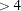) can be lengthy and computationally intensive. The computational time increases as
increases, whereis the number of increments ofandis the number of markers. For this reason, it is recommended that a model with several markers use consecutive grid searches with very few increments (small) in order to zero in on the correct values of.

The BTL model that is estimated is specified by the CROSS= option (backcross is the default) and the GEN= option (1 is the default). The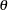parameters are calculated from the map data set by using whichever model is specified in the LINKMOD= option (Haldane is the default). Alternatively, a specific set ofvalues can be specified using the THETA option. A confidence interval of significance level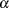can be requested by using the BOOT= option and specifying the number of bootstrap iterations.

You can specify the following options in the PARMEST statement.

ALPHA=number

specifies that a confidence level of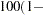number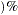is to be used in forming bootstrap confidence intervals for the penetrance parameters when the BOOT= option is given. This value of number must be between 0 and 1 and is set to 0.05 by default.

BOOT=number

requests that confidence intervals be calculated for the penetrance parameters by using number iterations of the bootstrap. You must input which recombination parameters (s) to use in the calculation by using the R option or an error is generated.

CROSS=BACK | B | DH
CROSS=INTER | F

specifies the type of cross for the input data set. The options include BACK or B for a backcross or, equivalently, DH for a doubled-haploid population. The other option is INTER or F for an F intercross. The default is backcross.

GEN=number

specifies the generation number of the offspring in the input data set. Valid values include any integer greater than or equal to one. The default is 1.

HETEROZYGOTE="heterozygote"
HE="heterozygote"

specifies the value for the heterozygous genotype used in the input data set. The default value is "B."

HOMOZYGOTE="homozygote"
HO="homozygote"

specifies the value for the homozygous genotype used in the input data set. The default value is "A." If the experimental design is an F cross, then this is the genotype homozygous for the parent 1 allele.

HOMOZYGOTE2="homozygote2"
HO2="homozygote2"

specifies the value for the genotype homozygous in the parent 2 allele used in the input data set. The default value is "C."

LINKMOD=HALDANE | H
LINKMOD=KOSAMBI | K

specifies the model to be used to calculate the marker recombination parameters from the marker location values in the map data set. The options include Haldane and Kosambi. The default value is Haldane.

LINKUNIT=CM | C
LINKUNIT=RECDIST | R

specifies the units used for the marker location variable in the marker data set. The options include centimorgans or recombination distance (kilobases). The default value is centimorgans.

OUTSTAT=SAS-data-set

names the SAS data set to be used for the parameter estimates and, when the BOOT= option is specified, confidence intervals.

PMAX=number

specifies the highest penetrance value that is considered in range and included in the output. Any real number is a valid value as long as it is greater than PMIN. The PMAX option is ignored if the R option, which precludes a grid search, is used. By default, there is no upper limit for the range of penetrance values included in the output.

PMIN=number

specifies the lowest penetrance value that is considered in range and included in the output. Any real number is a valid value as long as it is less than PMAX. The PMIN option is ignored if the R option, which precludes a grid search, is used. By default, there is no lower limit for the range of penetrance values included in the output.

R=number-list

specifies the values of(recombination parameters) used to estimate the penetrance parameters. There is onefor each of theadjacent marker/BTL pairs, whereis the number of markers in the MARKER statement. A list of values can be given to specify a differentfor each pair, or a single value can be specified to be used for all. If there are fewer thanvalues specified, the last value given is used for the remaining. If the R option is used to specify, the grid search parameters (RSTART, REND, and RINC) are ignored. The R option is required if the BOOT= option is specified. Theses are used to calculate the confidence intervals of the penetrance parameters in the bootstrap calculation. Eachmust be a real number greater than or equal to 0 and less than 0.5, and invalid values are replaced by the default value of 0.

REND=number

specifies the ending value for each recombination parameter in the grid search. The default value is 0.5. REND must be a real number greater than 0 and less than or equal to 0.5.

RINC=number

specifies the increment to be used for the recombination parameter grid search. The default value is 0.1. Any real number greater than 0 and less than or equal to 0.5 is valid.

RSTART=number

specifies the starting value for each recombination parameter in the grid search. The default value is 0. RSTART must be a real number from 0 to (but not including) 0.5.

SEED=number

specifies the initial seed for the random number generator used for creating the bootstrap samples when the BOOT= option is given. The value for number must be an integer; the computer clock time is used if the option is omitted or the integer specified is less than or equal to 0. For more details about seed values, see SAS Language Reference: Concepts.

THETA=number-list

specifies the values of recombination probabilities between adjacent pairs of markers listed in the MARKER statement. There is onefor each of the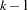pairs of adjacent markers, whereis the number of markers specified in the MARKER statement. A list of values can be given to specify a differentfor each pair, or a single value can be specified to be used for alls. If there are fewer thanvalues specified, the last value given is used for the remaining. Note:If the MAP= data set is specified and contains the variable Location, thevalues are calculated using these distances and this option is ignored. If locations are not provided in the MAP= data set and this option is omitted, then default values ofof 0.5 are used. Eachmust be a real number between 0 and 0.5, and invalid values are replaced by the default value of 0.5.

Note: This procedure is experimental.Previous Page | Next Page | Top of Page